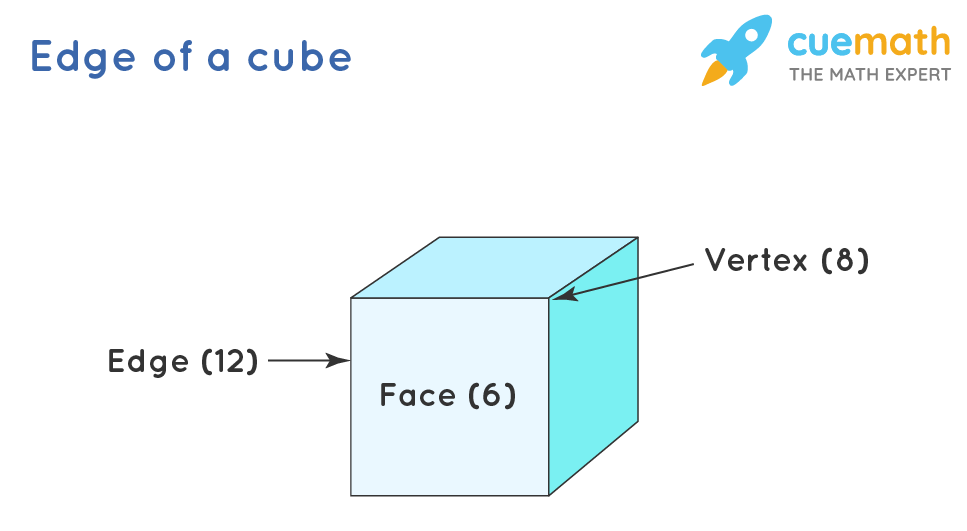# What Is the Edge of a Cube?

## Question: What is the edge of a cube?

A cube is a 3-dimensional figure with 12 edges, 6 faces, 8 vertices.

## Answer: The edge of any solid figure is the line segment joining the two vertices.

Let's understand the properties of cube in detail.

## Explanation:

A cube is a 3-d figure with 8 vertices. A line segment joining the two vertices is called an edge. There are 12 edges in a cube. All the 12 edges are of the same length in the cube.